Euler’s second law for 2-D motion

Two-dimensional motion: A three dimensional body is considered to be in two dimensional motion if the direction of its angular velocity does not change with time. If in an (x,y,z) coordinate system the direction of the angular velocity is in the z-direction, then for a two dimensional motion one can write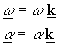where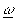and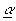are, respectively, the angular velocity and angular acceleration of the rigid body relative to an inertial frame. Each particle of a body in two-dimensional motion will be moving on a plane.

Angular momentum: There are several expressions for calculating angular momentum. First consider calculating the angular momentum relative to a fixed point O on an inertial reference frame. For this case one can use the relation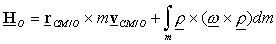whereis the position of dm relative to the center of mass.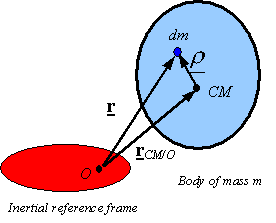To calculate the integral one needs to calculate its argument first. This can be done as follows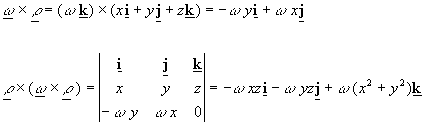Substituting into the above yields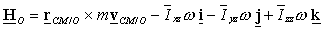whereare the products of inertia and moment of inertia about the center of mass.

It is easy to see by inspecting this equation that if one replaces the CM for point O,  that the angular momentum about the center of mass can be obtained as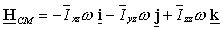If O is a point on the body which is fixed to the inertial reference frame (pin connected), then the moment of inertial of about O can be directly calculated as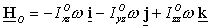where the products and moment of inertial are now calculated relative to axis passing through the fixed point O.Rate of change of angular momentum: When taking the time derivative to obtain the rate of change of angular momentum one needs to be in an inertial reference frame. Let  F be an inertial reference frame and B be the rigid body. If the (x,y,z) coordinate axis is fixed to the rigid body, the base vectors and the moments and products of inertial will be constant relative to the rigid body. Using the general kinematical rule for taking derivatives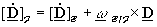one will obtainUsing the relation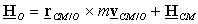one obtains the equation for the derivative with respect to time of the angular momentum with respect to a point O on an inertial frame as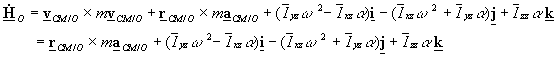If O is a point on the rigid body fixed to an inertial frame (pinned connected), then one will get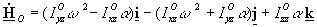Note: The products of inertia Ixz and Iyz are a measure of how balanced the body is. If the body is balanced, then the products of inertia will be zero. In case of a plate like body, where the z-coordinate of all points is zero, then the products of inertia will also be zero. In the case that the products of inertia are zero the rate of change of angular momentum will only have a z-component.

Euler’s 2nd law for 2-D motion: The following are some variations of Euler’s second law for a rigid body. They all follow from previously derived relations.

·        For any general two dimensional motion: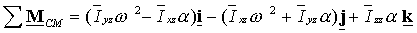·        For any general two dimensional motion where P is any arbitrary point and O is a point on an inertial reference frame: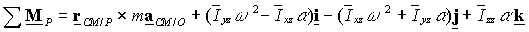·        For a rigid body pinned to an inertial frame at O: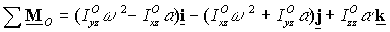Note: In general only the z-component of these equations are used. The other two components represent the moments needed to keep an unbalanced body in two dimensional motion (i.e., keep the direction of the angular velocity from changing). If the body is properly balanced so that the products of inertia are zero, then these components of the rate of change of angular momentum go to zero and, therefore, zero moment is needed to keep the body in two dimensional motion (i.e., if a balanced body is put in two dimensional motion, it will remain in two dimensional motion until moments are applied around the x- and/or y-axis to change this).